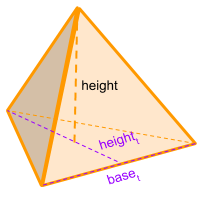Home » Polyhedra » Pyramids » Triangular Pyramid

# Volume of a Triangular PyramidVolume =
1/3
× Area base × Height

## Volume calculator for a triangular pyramid

Enter the height of the pyramid (height) and the area of the base (Area base) to get the volume automatically.

If you don't have the base area you can enter the height of the pyramid (height), the height of the triangle that forms the base of the pyramid (heighttt) to get the volume automatically.

## Description, how many faces, edges and vertices are there in a triangular pyramid

The triangular pyramid has 4 faces both the faces and the base are triangles, it has 4 vertices and 6 edges. If all the triangles that form the base and the other faces are exactly the same, then we are in presence of a tetrahedron, which has a simpler formula to calculate the volume.

## Formula for the volume of a triangular pyramid

To calculate the volume of a triangular pyramid we need to obtain the area of the base which is a triangle different than the other faces. Knowing the base's area, then you need to multiply this area by the height of the pyramid and divide it by 3.

Remember, in order to calculate the area of a triangle we need to know the base of the triangle, multiply it by the height of the triangle and finally divide it in two.
You can also use the online calculator to get the volume of the triangular pyramid automatically.

Volume =
1/3
× Area base × Height

Area base = Area triangle = (base t × height t) / 2

Share